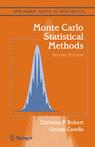## Typo in Bayesian Core [again]Reza Seirafi from Virginia Tech sent me the following email about Bayesian Core, which alas is pointing out a real typo in the reversible jump acceptance probability for the mixture model:

With respect to the expression provided on page 178 for the acceptance probability of the split move, I was wondering if the omission of the density of the auxiliary parameters u1, u2, and u3 — specially u2, since its density is not necessarily equal to 1 contrary to the other two — is a case of typo.

This is truly a typo, the acceptance probability at the bottom of page 178 should be$\min\left( \dfrac{\widetilde\pi_{(k+1)k}}{\widetilde\pi_{k(k+1)} }\,\dfrac{\varrho(k+1)}{\varrho(k)}\,\dfrac{\pi_{k+1}(\theta_{k+1})\ell_{k+1}(\theta_{k+1})}{pi(u_2)\pi_{k}(\theta_{k})\ell_{k}(\theta_{k})}\,\dfrac{p_{jk}}{(1-u_1)^2}\,\sigma_{jk}^2,1\right)$since u2 is indeed distributed from a non-uniform density. The physical reason for this (unacceptable!) typo is that I cut-and-pasted the LaTeX code from Monte Carlo Statistical Methods: (page 439) where u2 is a uniform variate! (Incidentally, there is a minor typo a few lines above: when defining$\mu_{(j+1)(k+1)} = \mu_{jk} - \dfrac{p_{j(k+1)}u_2}{p_{jk}-p_{j)(k+1)}}$,

it should be$\mu_{(j+1)(k+1)} = \mu_{jk} - \dfrac{p_{j(k+1)}u_2}{p_{jk}-p_{j(k+1)}}$,

another side casualty of cut-and-paste!) Obviously, this could also affect the associated R code but I checked it and found the line

jacob=(kprop-2)*log(1-propp[kprop]) - singleprior(propmix,kprop)

which I think is correcting for the normal proposal.

This typo will obviously be corrected in the next printing of Bayesian Core as well as in the new edition we plan to write in July. It also illustrates the folk theorem “One can never write the reversible jump acceptance probability right” that make me avoid using reversible jump each time I am facing a small enough collection of models to consider the exhaustive list of those models.

This site uses Akismet to reduce spam. Learn how your comment data is processed.